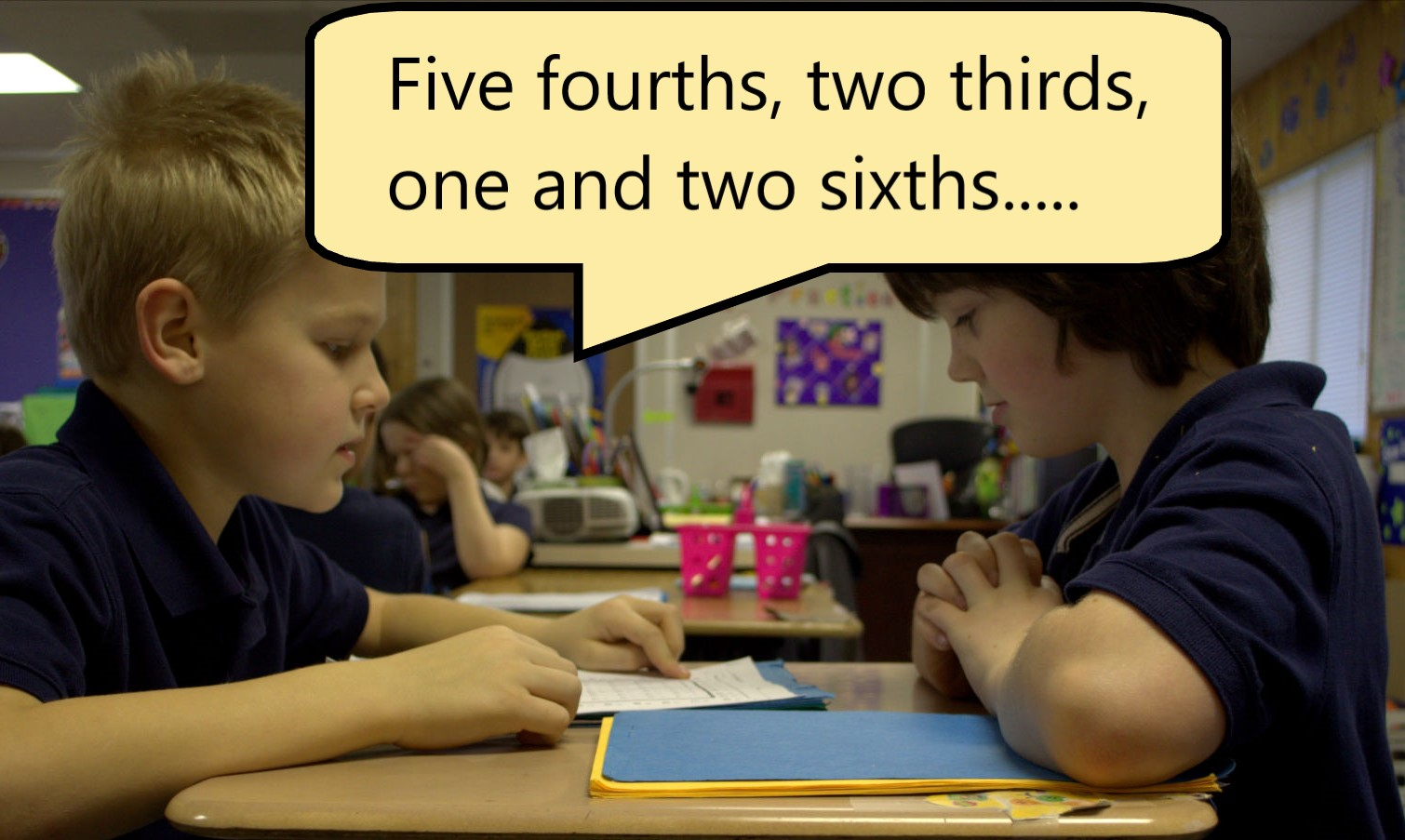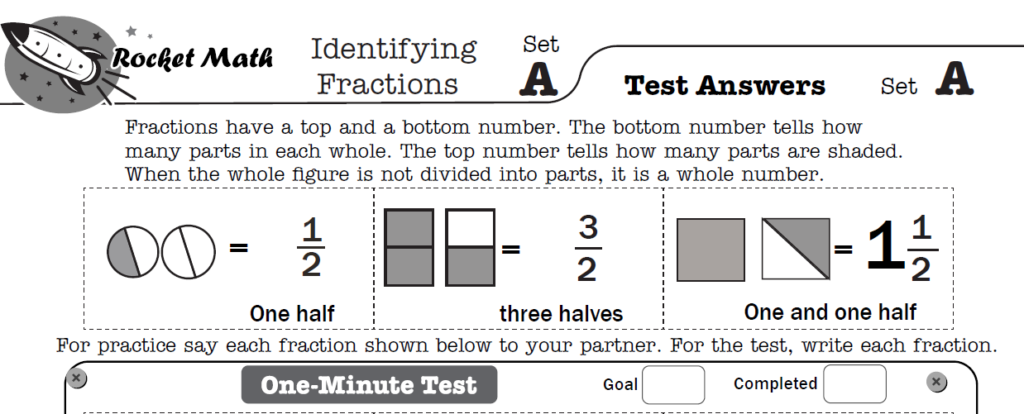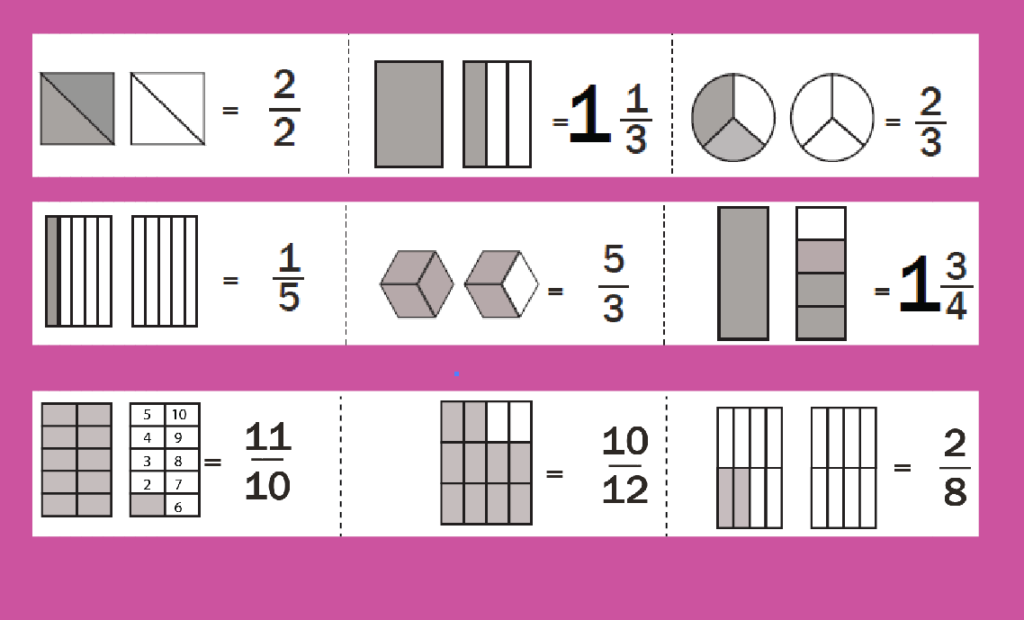Identifying Fractions: Creating fluent mathematicians

Fractions are unnecessarily hard for students to understand.  The reason?  Not enough practice with identifying a wide enough variety of fractions and ways of displaying them.  Rocket Math has introduced a great solution as part of the Universal Level Worksheet subscription–a new program called Identifying Fractions.

Identifying Fractions: Not just proper fractions anymore.One of the most powerful aspects of the Identifying Fractions program is that it is not limited to showing proper fractions.  Right from the beginning of Set A, students are introduced to improper fractions and mixed numbers.  They are taught the fundamental understanding that the bottom number tells you how many parts each whole is divided into.  At the same time, if the whole is not divided into parts, then we represent it as a whole number.  Finally, the top number tells how many parts are shaded (or used) regardless of whether that is more than 1 whole or less than one whole.  Identifying fractions that include improper fractions and mixed numbers from the beginning insures that students really understand fractions and don’t accidentally acquire the misrule that fractions are always less than one.

Identifying Fractions: Learn to speak their names also

Without a lot of oral practice students do not always know how to say the names of fractions.  Identifying fractions introduces three fractions in each set and includes the words for how to say them.  In the example here one half, three halves, and one and one half are written out at the top of the page.  This is all that is practiced as part of this first set.  This way, orally practicing with a partner means saying the names of the fractions, which are shown at the top of the page.  Students are not asked to say any fractions they haven’t seen written out first.

The fractions that students become familiar with include, halves, thirds, quarters, fifths, sixths, eighths, tenths and twelfths.  They see improper fractions and mixed number with every denominator.

Identifying Fractions: It’s not the shape that mattersWhen students don’t have a lot of practice with identifying fractions they may not see different shapes being divided into the same number of parts.  In Identifying Fractions we make a point of showing each fraction with at least three different shapes.  In this example you see thirds in a circle, in a cube shape, and as upright rectangles making a larger rectangle.  All of those are equally “thirds” because each whole figure is divided into three parts.  So there are three different shapes for halves, fourths, fifths and sixths.

By the time students are introduced to eighths, tenths and twelfths, they have already learned the rule that the shape doesn’t matter.

When students are eventually introduced to eighths, tenths and twelfths we don’t want to slow them down by having to laboriously count the number of parts in each figure.  As you can see to the left, the eighths are displayed as two sets of four rectangles on top of each other.  Eighths are always displayed that way, so they are easy to identify quickly.  Tenths are consistently displayed as two columns of five blocks with little numbers in them. (I know it’s a little weird, but it works to make them easily identifiable.) Twelfths are always shown as three sets of four rectangles on each other.  Students should notice these conventions so they can quickly identify the number of parts in those figures without having to count them.

Identifying Fractions:  Advanced students can fit in during Rocket Math routineIdentifying Fractions follows the standard Rocket Math routine.  Each student practices orally with the partner for a couple of minutes.  Then the two switch roles.  Finally everyone takes a 1 minute Daily Test.  A student in Identifying Fractions can be paired with any student in any other Rocket Math program as long as the student has an answer key to hand to their checker. Hopefully you remembered to print the answers on colored paper.

Unlike other Rocket Math programs, the test and the practice items are the same.  Of course, the students have a page without the answers, while their checker holds the answer key. Students practice by saying aloud to their partner the fractions shown in the test.  Then they take the test on those same items, but write the answer.

Identifying fractions has its own writing speed test, to be sure that student goals are individualized to their writing speed.  By the time students complete Set Z in this program they will have a strong understanding of fractions that will be fluent.  There are even 2-minute timings you can give every week or two for them to chart their progress as they get faster.  This is a great program for students of any grade from second grade on up who have finished the basics for their grade level.  It will really put them in good shape when dealing with fractions in later years.